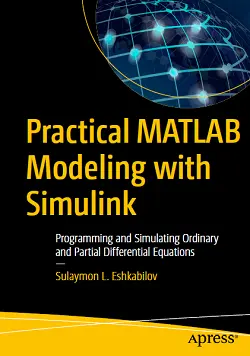# Practical MATLAB Modeling with Simulink### eBook Details:

• Paperback: 495 pages
• Publisher: WOW! eBook; 1st edition (April 8, 2020)
• Language: English
• ISBN-10: 1484257987
• ISBN-13: 978-1484257982

### eBook Description:

Practical MATLAB Modeling with Simulink: Programming and Simulating Ordinary and Partial Differential Equations

Employ the essential and hands-on tools and functions of MATLAB’s ordinary differential equation (ODE) and partial differential equation (PDE) packages, which are explained and demonstrated via interactive examples and case studies. This book contains dozens of simulations and solved problems via m-files/scripts and Simulink models which help you to learn programming and modeling of more difficult, complex problems that involve the use of ODEs and PDEs.

You’ll become efficient with many of the built-in tools and functions of MATLAB/Simulink while solving more complex engineering and scientific computing problems that require and use differential equations. Practical MATLAB Modeling with Simulink explains various practical issues of programming and modelling.

What You Will Learn

• Model complex problems using MATLAB and Simulink
• Gain the programming and modeling essentials of MATLAB using ODEs and PDEs
• Use numerical methods to solve 1st and 2nd order ODEs
• Solve stiff, higher order, coupled, and implicit ODEs
• Employ numerical methods to solve 1st and 2nd order linear PDEs
• Solve stiff, higher order, coupled, and implicit PDEs

After reading and using this book, you’ll be proficient at using MATLAB and applying the source code from the book’s examples as templates for your own projects in data science or engineering.

### 1 Response

1. April 16, 2020

[…] MATLAB and Simulink: From Novice to […]